Custom SearchSUMS Theorem 3. The derivative of the sum of two or more differentiable functions of x is equal to the sum of their derivatives. If two functions of x are given, such that u = g(x) and v = h(x) and also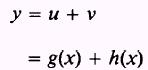thenPROOF. -andSubtract equation (5.1) from equation (5.2):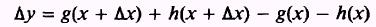Rearrange this equation such thatDivide both sides of the equation by Ax and then take the limit as: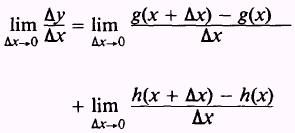But, by definitionandThen by substitution,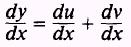EXAMPLE. Find the derivative of the functionSOLUTION: Theorem 3 indicates that we should find the derivative of each term and then show them as a sum; that is, ifandthenPRACTICE PROBLEMS: Find the derivative of the following:ANSWERS: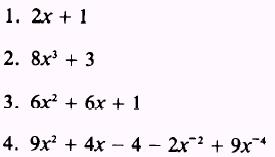Integrated Publishing, Inc. - A (SDVOSB) Service Disabled Veteran Owned Small Business